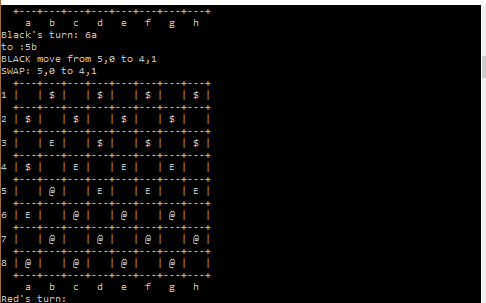Checkers Game using C++Posted by Samath10580 January 05, 2017

Checkers or Draughts is a group of strategy board games for two players which involve diagonal moves of uniform game pieces and mandatory captures by jumping over opponent pieces. Draughts or checkers is played by two opponents, on opposite sides of the gameboard. One player has the dark pieces; the other has the light pieces. Players alternate turns. A player may not move an opponent's piece. A move consists of moving a piece diagonally to an adjacent unoccupied square. If the adjacent square contains an opponent's piece, and the square immediately beyond it is vacant, the piece may be captured (and removed from the game) by jumping over it.Code:

```#include<stdio.h>

#define ROWS 8
#define COLS 8

#define EMPTY 1
#define RED 2
#define BLACK 3
#define REDKING 4
#define BLACKKING 5

#define ISRED(c)  (c == RED || c == REDKING)
#define ISBLACK(c) (c == BLACK || c == BLACKKING))
#define ISEMPTY(c) (c == 1)

void printDisplay(int d[][COLS]);
void swapIJKL(int d[ROWS][COLS], int i, int j, int k, int l);
char value2symbol(int i);
void printDisplayFancy(int d[][COLS]);
int Playersturn(int d[][COLS], int player,int i,int j,int k,int l);

void printDisplayFancy(int d[][COLS])
{
int rr, cc, pp;

printf("  +---+---+---+---+---+---+---+---+\n");

for (rr=0; rr<ROWS; ++rr)
{
printf("%d |", rr+1);
for (cc=0; cc<COLS; ++cc)
{
printf(" %c |", value2symbol(d[rr][cc]) );
}
printf("\n");
printf("  +---+---+---+---+---+---+---+---+\n");
}

printf("    a   b   c   d   e   f   g   h\n");
}

void swapIJKL(int d[ROWS][COLS], int i, int j, int k, int l)
{
int temp;

printf("SWAP: %d,%d to %d,%d\n", i, j, k, l);

temp = d[i][j];

d[i][j] = d[k][l];

d[k][l] = temp;
}

char value2symbol(int i)
{
switch(i)
{
case 0:
return ' ';
case 1:
return 'E';
case 2:
return '\$';
case 3:
return '@';
}
return ('?');
}

int Playersturn(int d[][COLS], int player,int i,int j,int k,int l)
{
int jmp_r;
int jmp_c;

if(player == RED){
printf("RED move from %d,%d to %d,%d\n", i, j, k, l);
} else {
printf("BLACK move from %d,%d to %d,%d\n", i, j, k, l);
}

if(i < 0 && ROWS <= i){
printf("i is out of bounds\n");
return -1;
}
if(j < 0 && COLS <= j){
printf("j is out of bound");
return -1;
}

if(k < 0 && ROWS <= k){
printf("k is out of bounds");
return -1;

}
if(l < 0 && COLS<= l){
printf("l is out of bounds\n");
return -1;
}

if(player == RED){
if(d[i][j] != RED){
return -1;
}
} else {
if(d[i][j] != BLACK){
return -1;
}
}

if(d[k][l] != EMPTY){
printf("You must move to a empty location");
return -1;
}

if(player == RED){
if(i >= k){
printf("RED player must move down\n");
return -1;
}
} else {
if(i <= k){
printf("BLACK player must move up\n");
return -1;
}
}

if(i - k == -1 || i - k == 1){
if(j - l == 1 || j - l == -1){
swapIJKL(d,i,j,k,l);
return 0;
}
}
if(i - k == -2 || i - k == 2){
if(j - l == -2 || j - l == 2){
if(i < k){
jmp_r = i + 1;
} else {
jmp_r = i - 1;
}
if(j < l){
jmp_c = j + 1;
} else {
jmp_c = j - 1;
}

if(player==RED && d[jmp_r][jmp_c]!=BLACK)
{
printf("Enemeny is not Black at %d%d",jmp_r, jmp_c);
return -1;
}
if(player==BLACK && d[jmp_r][jmp_c] != RED){
printf("you can only jump over an enemy player\n");
return -1;
}

d[jmp_r][jmp_c] = 1;
swapIJKL(d,i,j,k,l);
return 0;
}
}

printf("You can only move diagnally\n");
return -1;

}

int main()
{
int r,c;
int pr, pb;
int i, k;
char j, l;
int d[ROWS][COLS]={
{0,2,0,2,0,2,0,2},
{2,0,2,0,2,0,2,0},
{0,2,0,2,0,2,0,2},
{1,0,1,0,1,0,1,0},
{0,1,0,1,0,1,0,1},
{3,0,3,0,3,0,3,0},
{0,3,0,3,0,3,0,3},
{3,0,3,0,3,0,3,0}};

for(;;){
printDisplayFancy(d);
while(1){
printf("Red's turn: ");
scanf("%d%c",&i,&j);
printf("to: ");
scanf("%d%c",&k,&l);
if(Playersturn(d, RED, i-1,j - 'a',k-1,l - 'a') == 0)
break;
printf("Illegal move, try again\n");
}

printDisplayFancy(d);
while(1){
printf("Black's turn: ");
scanf("%d%c",&i,&j);
printf("to :");
scanf("%d%c",&k,&l);
if(Playersturn(d, BLACK, i-1,j - 'a',k-1,l - 'a') == 0)
break;
printf("Illegal move, try again\n");
}
}

getchar();
getchar();
return 0;
}

```